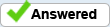# Field validation currency

I have 4 fields that take a money value, a 5th field totals the 4 fields.

I then have another field where you enter the maximum allowed cost.

If the max cost is less than the total cost, generate a message. I have this but is not working correctly. I hear it is because the fields are formatted as currency?

var ex1 = this.getField("Totalcost").value;
var ex2 = this.getField("Maximumcost").value;

if (ex2 < ex1) {
app.alert('The "Maximum Per Trip Cost" must equal or exceed the "Total Cost Estimate." ');

getField("Maximumcost").setFocus();
}

Murray James